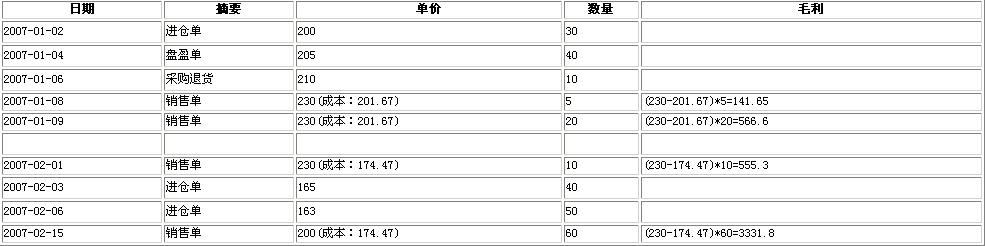# 进销存设计与分析_成本核算(7)

采购进仓单、采购退货单、盘点单(盈、亏)

1、把所有的单据合成一个主表和从表，用主表做标识区分单据：采购订单、采购退货、采购进仓单、盘点单、商品调拨单、销售订单、销

2、在从表增加一个字段：成本单价，只用于销售单和销售退货单
3、把所有单据主从表合并，有利于编程开发

(1)、成本=进仓单+盘点单(盘盈)-盘点单(盘亏)-采购退货
(2)、把成本的结果写入单据从表的“成本单价”
(3)、由于是按月成本核算，当计算本月的成本时，要把上个月的结转到本月来核算2007-01月的成本计算：

成本单价=(200*30+205*40-210*10)/(30+40-10)=(6000+8200-2100)/60=201.67

结存余额=200*30+205*40-210*10-201.67*(20+5)=7058.25；结存数量=30+40-10-5-20=35

2007-02月的成本计算：

成本单价=(上个月的结存余额+本月的进项金额)/(上个月的结存数量+本月进项数量)
=(7058.25+165*40+163*50)/(35+40+50)=(7058.25+6600+8150)/125=174.47

1、接上一次核算主要判断单据从表的字段“成本单价”是否为空就可以了
2、全面核算，是把所有的单据(采购退货、采购进仓单、盘点单)重新核算一下成本